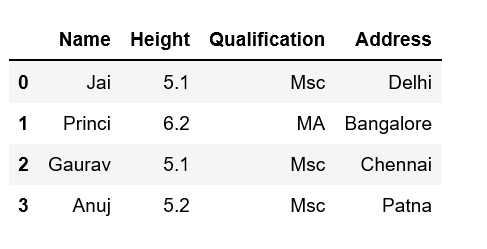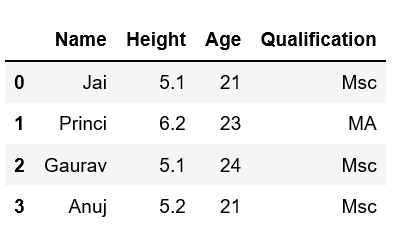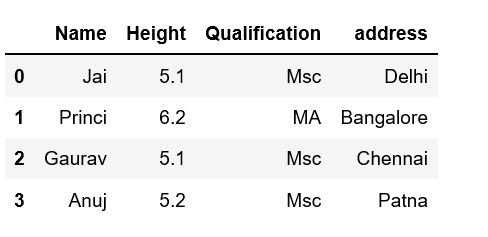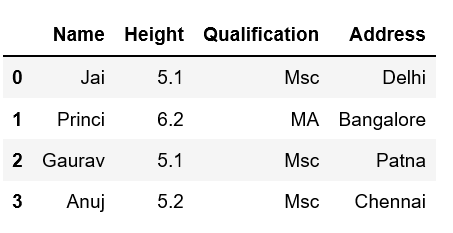# Adding new column to existing DataFrame in Pandas

• Difficulty Level : Easy
• Last Updated : 09 Jun, 2022

Let’s discuss how to add new columns to the existing DataFrame in Pandas. There are multiple ways we can do this task.Method #1: By declaring a new list as a column.

## Python3

 `# Import pandas package` `import` `pandas as pd`   `# Define a dictionary containing Students data` `data ``=` `{``'Name'``: [``'Jai'``, ``'Princi'``, ``'Gaurav'``, ``'Anuj'``],` `        ``'Height'``: [``5.1``, ``6.2``, ``5.1``, ``5.2``],` `        ``'Qualification'``: [``'Msc'``, ``'MA'``, ``'Msc'``, ``'Msc'``]}`   `# Convert the dictionary into DataFrame` `df ``=` `pd.DataFrame(data)`   `# Declare a list that is to be converted into a column` `address ``=` `[``'Delhi'``, ``'Bangalore'``, ``'Chennai'``, ``'Patna'``]`   `# Using 'Address' as the column name` `# and equating it to the list` `df[``'Address'``] ``=` `address`   `# Observe the result` `print``(df)`

Output:Note that the length of your list should match the length of the index column otherwise it will show an error.

Method #2: By using DataFrame.insert()

It gives the freedom to add a column at any position we like and not just at the end. It also provides different options for inserting the column values.

Example

## Python3

 `# Import pandas package ` `import` `pandas as pd`   `# Define a dictionary containing Students data` `data ``=` `{``'Name'``: [``'Jai'``, ``'Princi'``, ``'Gaurav'``, ``'Anuj'``],` `        ``'Height'``: [``5.1``, ``6.2``, ``5.1``, ``5.2``],` `        ``'Qualification'``: [``'Msc'``, ``'MA'``, ``'Msc'``, ``'Msc'``]}`   `# Convert the dictionary into DataFrame` `df ``=` `pd.DataFrame(data)`   `# Using DataFrame.insert() to add a column` `df.insert(``2``, ``"Age"``, [``21``, ``23``, ``24``, ``21``], ``True``)`   `# Observe the result` `print``(df)`

Output:Method #3: Using Dataframe.assign() method

This method will create a new dataframe with a new column added to the old dataframe.

Example

## Python3

 `# Import pandas package` `import` `pandas as pd`   `# Define a dictionary containing Students data` `data ``=` `{``'Name'``: [``'Jai'``, ``'Princi'``, ``'Gaurav'``, ``'Anuj'``],` `        ``'Height'``: [``5.1``, ``6.2``, ``5.1``, ``5.2``],` `        ``'Qualification'``: [``'Msc'``, ``'MA'``, ``'Msc'``, ``'Msc'``]}`     `# Convert the dictionary into DataFrame` `df ``=` `pd.DataFrame(data)`   `# Using 'Address' as the column name and equating it to the list` `df2 ``=` `df.assign(address``=``[``'Delhi'``, ``'Bangalore'``, ``'Chennai'``, ``'Patna'``])`   `# Observe the result` `print``(df2)`

Output:Method #4: By using a dictionary

We can use a Python dictionary to add a new column in pandas DataFrame. Use an existing column as the key values and their respective values will be the values for a new column.

Example

## Python3

 `# Import pandas package` `import` `pandas as pd`   `# Define a dictionary containing Students data` `data ``=` `{``'Name'``: [``'Jai'``, ``'Princi'``, ``'Gaurav'``, ``'Anuj'``],` `        ``'Height'``: [``5.1``, ``6.2``, ``5.1``, ``5.2``],` `        ``'Qualification'``: [``'Msc'``, ``'MA'``, ``'Msc'``, ``'Msc'``]}`   `# Define a dictionary with key values of` `# an existing column and their respective` `# value pairs as the # values for our new column.` `address ``=` `{``'Delhi'``: ``'Jai'``, ``'Bangalore'``: ``'Princi'``,` `           ``'Patna'``: ``'Gaurav'``, ``'Chennai'``: ``'Anuj'``}`   `# Convert the dictionary into DataFrame` `df ``=` `pd.DataFrame(data)`   `# Provide 'Address' as the column name` `df[``'Address'``] ``=` `address`   `# Observe the output` `print``(df)`

Output:My Personal Notes arrow_drop_up
Recommended Articles
Page :